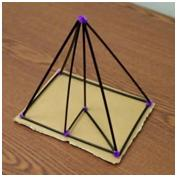# Volume and Surface Area Webpage

Prisms, Pyramids, Cylinders, and Cones

The Ultimate Goal: Find the Volume and Surface Area of Prisms, Pyramids, Cylinders, and Cones*

Finding the volume and surface area of prisms and pyramids is particularly interesting, and worthwhile for students, because it involves use of the Pythagorean theorem, modeling, visualization, measurement, and problem solving.

Here are some links to help see what's going on.

## My Flowchart

Here is my flowchart (.doc) showing a possible progression for teaching these concepts.

## The Key is Understanding the Rectangular Pyramid

In my humble opinion: A big key to understanding volume and surface area is to understand the rectangular pyramid. It has three key “heights” and three key lengths on the rectangular base. This handout describes the "Essential Tetrahedron," whose 4 faces are all right triangles (made to order for the Pythagorean Theorem)!!

There is an Essential Tetrahedron for every pyramid with a polygonal base.

### How to Build a 3D Model of a Pyramid

Click here for the steps on how to build a rectangular pyramid out of coffee stirrers (or straws) and fuzzy sticks (or chenille stems). (Click here for the steps on how to build a rectangular or square pyramid.)

Here's a video:## Using Geogebra

worksheet.html

Online worksheet investigating volume and surface area of cylinders + answers

Online worksheet investigating volume and surface area of rectangular pyramids + answers

.html

Online worksheet investigating volume and surface area of cones + answers

Online worksheet investigating volume of triangular prisms + answers

## From Wolfram Demonstrations Project

Square Pyramid and Its Right Triangles (especially #2, 3, 4, 8) from Wolfram Demonstrations Project: http://demonstrations.wolfram.com/SquarePyramidAndItsRightTriangles/

Three Pyramids That Form a Cube from Wolfram Demonstrations Project: http://demonstrations.wolfram.com/ThreePyramidsThatFormACube/

## Other

Miscellaneous volume and surface area worksheets online